#### How many numbers are in this image?themindsjournal.com

There are actually 10, if you look hard enough.funwithpuzzles.com

#### Which image is different?BRiddles

Look at the eyes.Daily Mail

The word "the" is printed twice. Oops.shakethebrain.com

Look closely; lines cut off several of the boxes, preventing them from filling.

#### Count the matchsticks in this picture.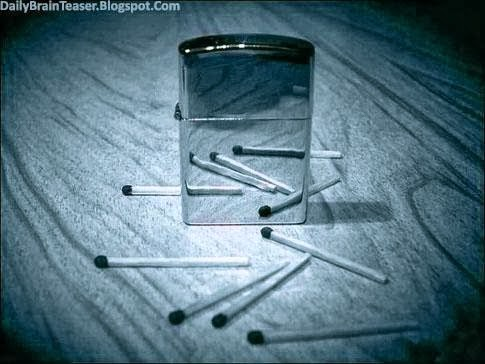The lighter is metallic; don't count the matchsticks in the reflection.

#### How many triangles are in this picture?Scoopnest.com

16 + (8x2) + (4x2) + (1x2) + (1x2) = 40.

#### What number should replace the question mark?Gpuzzles

The numbers in each row and column add up to 15.

#### This one's a freebie. Can you find the soccer ball?Gergely Dudas/Dudolf

Starting at the flags, go up two rows and slowly look over every figure towards the right side.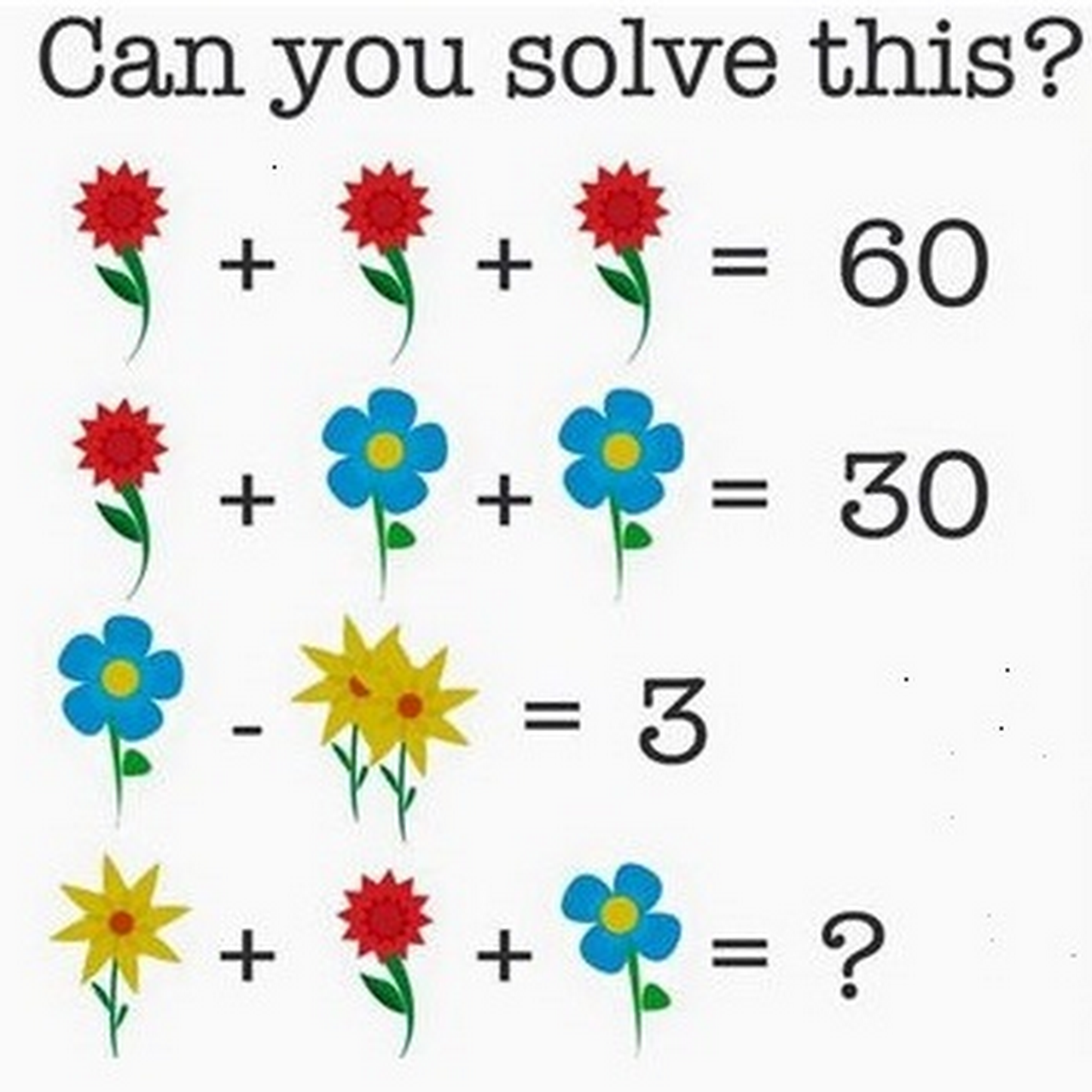BRiddles

Pretend that the flowers are just "x," "y," and "z," and this gets much easier. The first equation tells us that a red flower (x) is 20. Solving the second one shows that a blue flower (y) is 5. Solving the third equation shows that yellow flowers (z) are worth 1 each. The final equation is x+y+z, which is 5+20+1; however, note that the blue flower only has four petals. Subtract one for the missing pedal, and you get 25.

#### How many triangles are in this picture?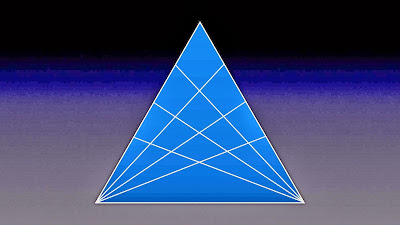BRiddles

Again, you've got to count every single triangle—even when they overlap.

#### How many squares are on a chess board?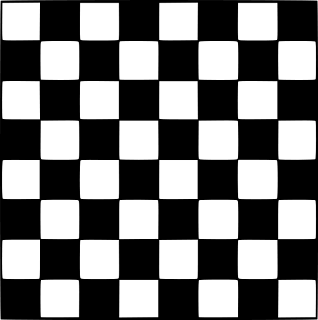BRiddles

Again, you've got to count every square, including squares that encompass the other squares.

#### This one's a classic.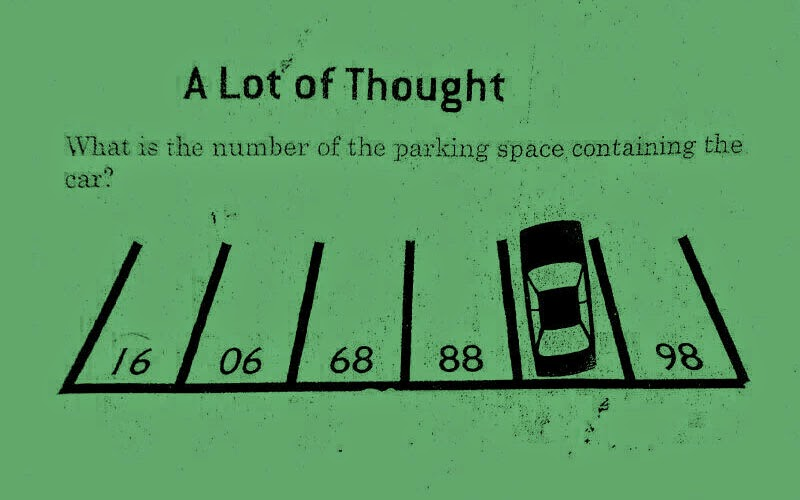BRiddles

Invert the picture, and the answer is obvious.Depositphotos

Look closely at the bear's bow tie.

#### Which is the correct silhouette for the rooster?mentalup.co

Hey, some of these are easy.

#### If you folded up this cube, which picture would it create?BRiddles

It's C, but it took us a while to figure that out.

#### Which two of these cats are completely identical?BRiddles

Look closely at the collar, eyes, whiskers, tail, and thigh to spot the differences.

#### What number should replace the question mark?BRiddles

Center Number = (Top Left Number * Bottom Left Number)/(Top Right Number * Bottom Right Number)

#### What would the scale in the final picture read?FunWithPuzzles.com

The dog weighs 17 kilograms, while the cat weighs 7 kilograms and the rabbit weighs 3 kilograms. Add it all up, and you've got the answer.FunWithPuzzles.com

It's C. This one's not a trick, but it takes a while to figure out.

#### By only touching one glass, you need to make the row alternate between empty and full glasses. What glass do you touch?Daily Mail

Take the second glass from the left, then pour it into the empty glass second from the right.

#### How many squares are in this picture?Daily Mail

There are 40 squares. Remember, don't count rectangles.

#### What option fits best with the missing column?BRiddles

In each column, the order is reversed from the previous column while the lowest digit is omitted. Logically, option D works best.

#### How many triangles are in this picture?BRiddles

There are 24 total.

#### What number should replace the question mark?BRiddles

#### How many squares are in this picture?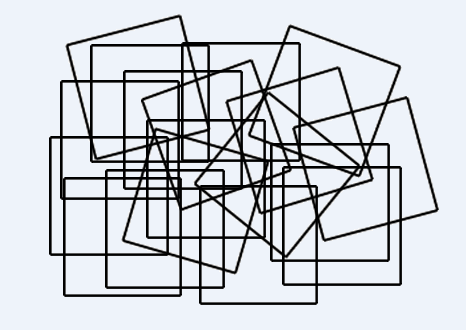BRiddles

Okay, this was a tough one. There's no trick, you just need to be careful.

#### Which switch turns on the light?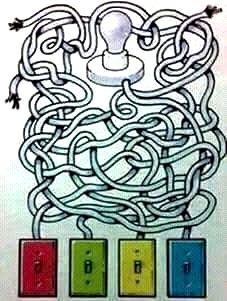moillusions.com

Yeah, it's frustrating, but trace them back, and it's clearly the yellow switch.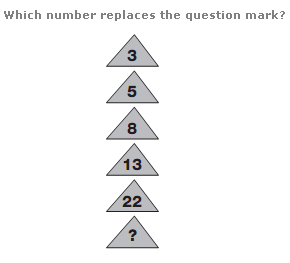PuzzleFry

3*2 – 1 = 5, 5*2 – 2 = 8, 8*2 – 3 = 13, 13*2 – 4 = 22, so 22*2 – 5 = 39.

#### What number comes next in this sequence?Mentalfeed

Each number is the sum of the previous two numbers.

#### 10 cows eat 10 bales of hay in 10 hours. How many hours does it take 20 cows to eat 20 bales of hay?iStock.com/Kamisoka

Each cow takes 10 hours to eat its bale of hay.

#### If you fold these pieces of paper, which one resembles the cube shown?funwithpuzzles.com

B and C don't fit, and D creates a mirrored image of the cube—not the original pattern.

#### Which square comes next?funwithpuzzles.com

Each square exchanges the opposite squares of the previous square.

#### Which of these sets of matchsticks doesn't fit with the others?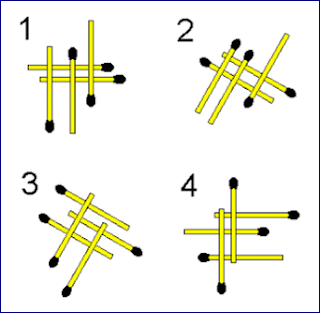Briddles

The third set doesn't form two full rectangles; all of the others do.

#### What's the solution to this equation?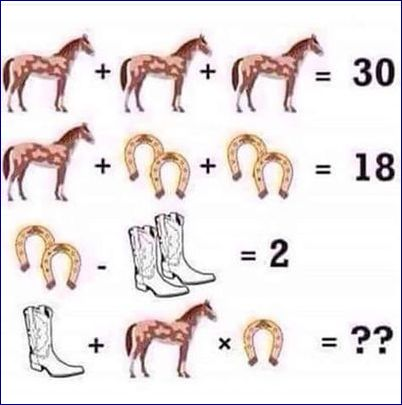BRiddles

Solve the first equations, and you'll find that the horse is worth 10, the boot is worth 1, and the horseshoe is worth 2.

#### What month is represented in this picture?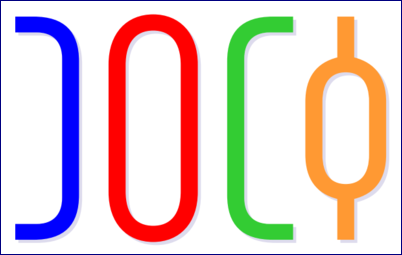BRiddles

Cut the image in half; the bottom half says "July."

#### What letter should replace the question mark in the last circle?BRiddles

Give the letter in the center of each circle a numerical value (A=1, B=2, and so on). The letters in each circle advance according to that number. With that in mind, T is the right answer.

#### Could this arrangement of pieces occur in a real game of chess?BRiddles

White could have started the game by moving their knight up, then moving it back after Black moved their pawn.

#### What number should replace the question mark?BRiddles

In each row, take the first number times the second number, then add it to the sum of the first number minus the second number.

#### How many triangles are in this picture?BRiddles

By this point, you're pretty good at counting triangles.

#### Which clock is wrong?BRiddles

"VIII" is where the "VII" should be.